Deep Learning

# Neural-Symbolic Regression: Distilling Science from Data

July 20, 2022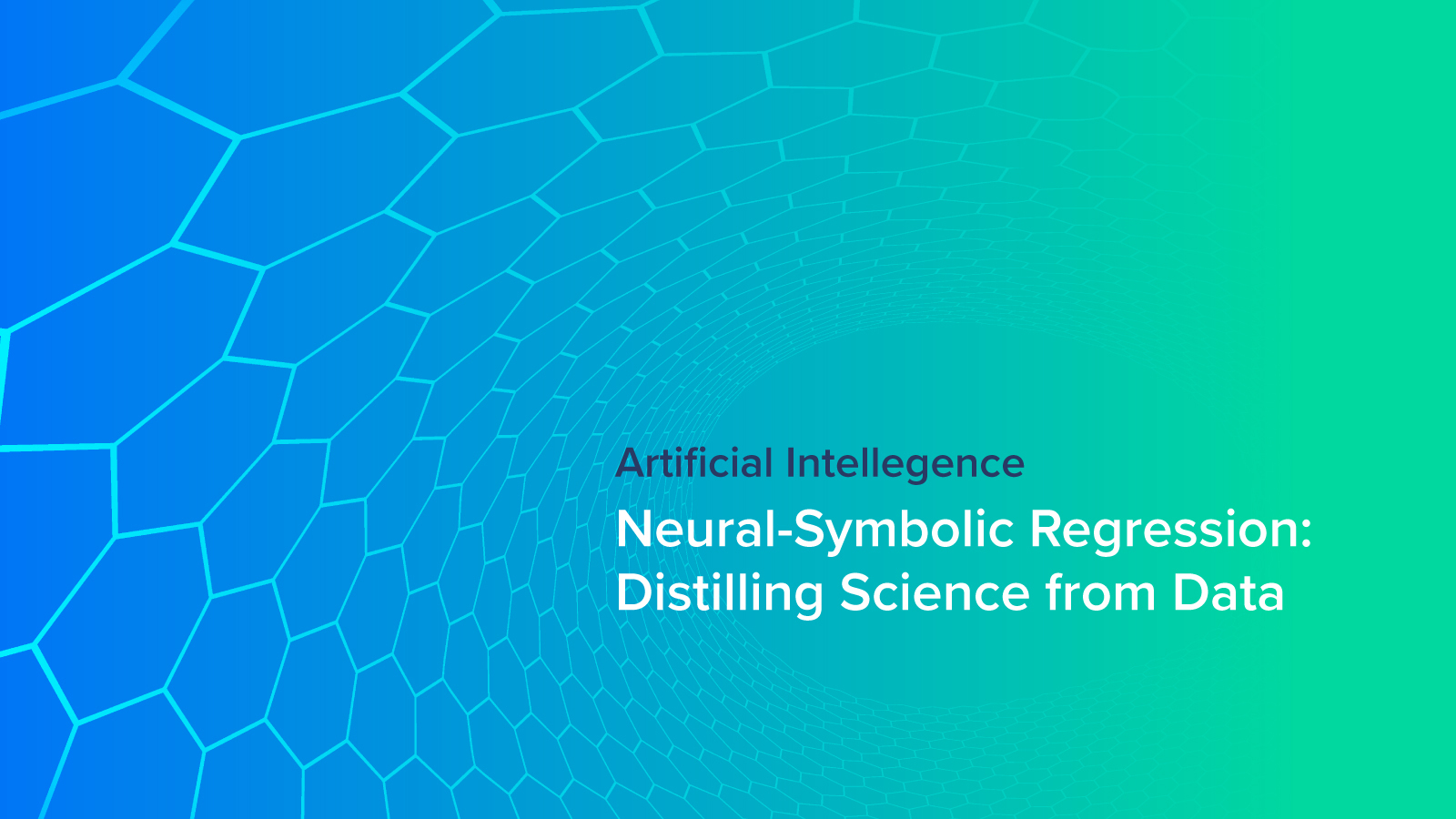The universe is noisy and confusing, complex enough to make predictions difficult. Human intelligence and intuition facilitate a basic understanding of some of the activities of the world around us. And they do so well enough to make basic sense of events at the macro space and time scales of the limited perspectives of individuals and small groups.

The natural philosophers of human prehistory and early history were mostly limited to common sense rationalization and guess and check. The limitations of these methods, especially for things that are just too big or too complex, are readily apparent in the prevalence and influence of superstition and magical thinking.

Not to disparage guessing and checking (it is the basis for the modern scientific method) but to see that a change in the human capability to investigate and understand was kindled by the desire and tools to distill physical phenomena into mathematical expressions.

This was especially evident after the time of Newton and others leading to the enlightenment, though there were traces of analytical reductionism in antiquity as well. The ability to go from observations to mathematical equations (and the predictions made possible by those equations) is an integral part of scientific exploration and progress.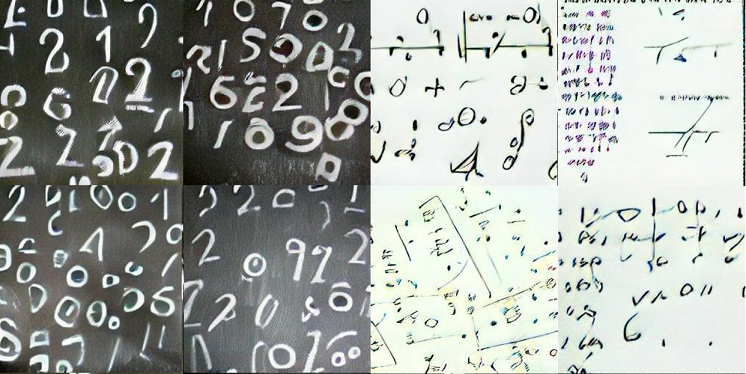Deep learning is also fundamentally about learning transformations relating to input-output observations, just like human scientists attempting to learn functional relationships between inputs and outputs in the form of mathematical expressions.

The difference, of course, is that the input-output relationship learned by a deep neural network (a consequence of the universal approximation theorem) consists of an un-interpretable “black box” of numerical parameters, primarily weights, biases, and the nodes they connect.

The universal approximation theorem states that a neural network fulfilling very lenient criteria should be able to closely approximate any well-behaved function. In practice, neural networks are a brittle and leaky abstraction when representing input-output relationships arising from simple and precise underlying equations.

Neural networks tend to perform very poorly when making predictions outside of the distribution they were trained on unless special care is taken to train a model (or model ensemble) to predict uncertainty.

Deep learning predictions also don’t fare too well at making falsifiable predictions, i.e. the hypotheses making up the foundation of the scientific method, out of the box. So while deep learning is a well-proven tool adept at fitting data, its utility in arguably one of the most human pursuits of all, exploration of the universe around us via the scientific method, has been limited.

Despite the various shortcomings of deep learning in the human endeavor of science, we would be foolish to disregard the tremendous fitting power and numerous successes of deep learning in science-based disciplines.

Modern science generates tremendous amounts of data unfeasible for an individual (or even a small group) to look at the output and make intuitive leaps from noisy data to clean mathematical equations.

For this, we turn to symbolic regression, the automated or semi-automated method of reducing data to equations.

### The Current Gold Standard: Evolutionary Methods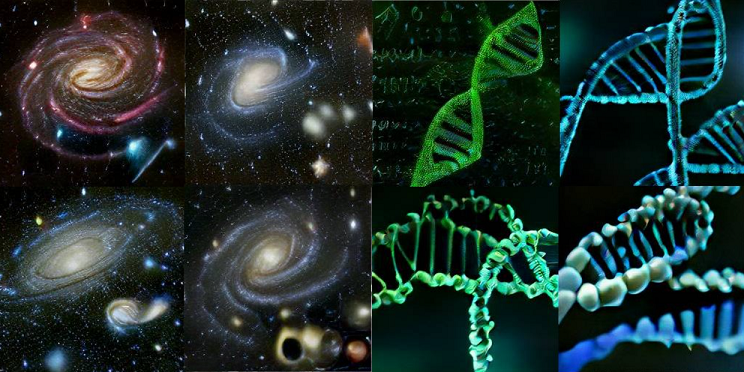Before we get into some exciting recent research in applying modern deep learning to symbolic regression, we have to first visit the current state of the art of evolutionary methods for turning datasets into equations. The most commonly mentioned symbolic regression software package is Eureqa, based on genetic algorithms.

Eureqa was originally developed as a research project at Cornell University in Hod Lipson’s group, and made available as proprietary software from Nutonian, later acquired by DataRobot. Eureqa is integrated into the Datarobot platform, under Eureqa co-author and CTO of Datarobot Michael Schmidt.

Eureqa and similar symbolic regression tools use genetic algorithms to simultaneously optimize a population of equations for accuracy and simplicity.

TuringBot is an alternative symbolic regression package based on simulated annealing. Simulated annealing is an optimization algorithm analogous to the metallurgical annealing used to alter the physical properties of metals.

In simulated annealing, candidate solutions to an optimization problem are chosen with a decreasing “temperature,” where higher temperatures correspond to accepting worse solutions and are used to facilitate exploration early on, enabling the search for a global optimum and providing the energy to escape local optima.

TuringBot is available as a free version, but there are significant limits on dataset size and complexity and the code is not open for modification.

While commercial symbolic regression software (especially Eureqa) provides important baselines for comparison when developing new tools for symbolic regression, the utility of closed-source programs is limited.

An open-source alternative, called PySR, released under the Apache 2.0 license and led by Princeton Ph.D. student Miles Cranmer, shares the optimization objectives of accuracy and parsimony (simplicity) as well as combining methods used by Eureqa and TuringBot.

As well as providing a free and freely modifiable software library for performing symbolic regression, PySR is interesting from a software perspective: it is written in Python but uses the Julia programming language as a speedy backend.

While genetic algorithms are generally considered the current state-of-the-art for symbolic regression, there has been an exciting explosion of new symbolic regression strategies in the last few years.

Many of these new developments take advantage of the modern deep learning models, either as a function-approximation component in a multi-step process, or in an end-to-end manner based on large transformer models, originally developed for natural language processing, and anything in between.

In addition to new symbolic regression tools based on deep learning, there has been a resurgence in probabilistic and statistical methods as well, especially Bayesian statistics.

Combined with modern computational capabilities, the new crop of symbolic regression software is not only interesting research in its own right but provides real utility and contribution to scientific disciplines embracing big datasets and comprehensive experiments.

### Symbolic Regression with Deep Neural Networks as Function Approximators

Thanks to the Universal Approximation Theorem, described and investigated by Cybenko and Hornik in the late 1980s/early 1990s, we can expect neural networks with at least one hidden layer of non-linear activations to be able to approximate any well-behaved mathematical function.

In practice, we tend to get much better performance with much deeper neural networks on more complex or complicated problems. However, in principle, a single hidden layer is all you need to approximate a wide range of functions.

The physics-inspired AI Feynman algorithm exploits the universal approximation theorem as one piece in a much more complex puzzle.

AI Feynman (and its successor, AI Feynman 2.0) were developed by physicists Silviu-Marian Udrescu and Max Tegmark (along with some colleagues). Reflecting the background of the authors, AI Feynman takes advantage of functional properties found in many physics equations, such as smoothness, symmetry, and compositionality along with a handful of others.

Neural networks come into play as function approximators, learning the input-output transform pair represented in a dataset (or “mystery,” as they call them) and facilitating the investigation of these properties by producing synthetic data under the same functional transform.

The functional properties AI Feynman leverages to solve problems are common in equations from physics but are not arbitrarily applied to the space of all possible mathematical functions. However, they are still reasonable assumptions to look for in a wide variety of functions corresponding to the real world.

As in the genetic algorithm and simulated annealing approaches described earlier, AI Feynman fits each new dataset from scratch. There is no generalization or pre-training involved and the deep neural networks make up only one carefully orchestrated piece of a much larger, physics-informed system.

AI Feynman symbolic regression made an impressive showing in deciphering a set of 100 equations (or mysteries) from The Feynman Lectures on Physics, but the lack of generalization means that each new dataset (corresponding to a new equation) requires a substantial computational budget.

A new cohort of deep learning strategies for symbolic regression utilize the wildly successful transformer model family, originally introduced as natural language models by Vaswani et al. These new approaches aren’t perfect, but the utilization of pre-training can make for substantial computational savings at inference time.

### First-Generation Symbolic Regression Based on Natural Language Models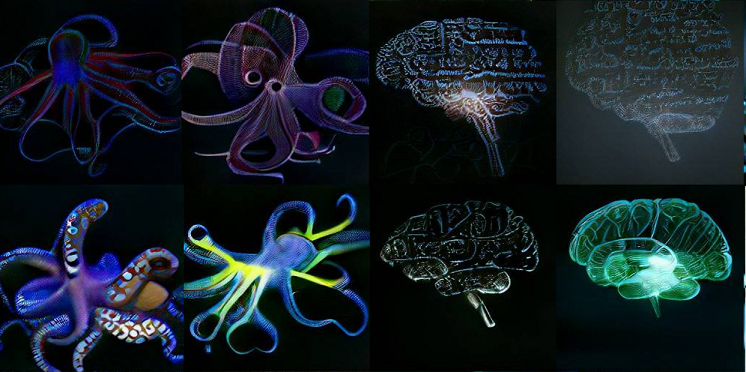Given the massive success of extremely large attention-based transformer models on diverse tasks in computer vision, audio, reinforcement learning, recommender systems, and many other domains (in addition to the original role of text-based natural language processing), it’s no surprise that transformer models would eventually be applied to symbolic regression as well.

While the domain of numerical input-output pairs to symbolic sequences requires some careful engineering, the sequence-based nature of mathematical expressions naturally lends itself to the transformer approach.

Crucially, the use of transformers for generating mathematical expressions enables them to take advantage of pre-training on the structure and numerical meaning of millions of automatically generated equations.

This also lays the foundations for improving models by scaling larger. Scaling is one of the principal advantages of deep learning, where larger models and more data continue to improve model performance far beyond the classical statistical learning limits of over-fitting.

Scaling was the principal advantage of a paper by Biggio et al. entitled “Neural-Symbolic Regression that Scales,” which we’ll refer to as NSRTS. The NSRTS transformer model uses a specialized encoder to cast each dataset of input-output pairs into a latent space. The encoded latent space has a fixed size, regardless of the input size to the encoder.

The NSRTS decoder then builds a sequence of tokens to represent an equation, conditioned on the encoded latent space and the symbols generated so far. Crucially, the decoder only outputs placeholders for numerical constants, but otherwise uses the same vocabulary as the pre-training equations dataset.

NSRTS uses PyTorch and PyTorch Lightning and is available under a permissive open-source MIT License.

Following the generation of a constant-free equation, referred to as an equation skeleton, NSRTS uses gradient descent to optimize constants. This approach, which layers a generic optimization algorithm on top of sequence generation, is shared by the so-called “SymbolicGPT,'' developed contemporaneously by Valipour et al.

Instead of an attention-based encoder as in the NSRTS approach, Valipour et al. used a model loosely based on Stanford’s point-cloud model, PointNet, to generate a fixed-dimension set of features for a transformer decoder to use for generating equations. Like NSRTs, SymbolicGPT uses BFGS to find numerical constants for equation skeletons that the transformer decoder generates.

### Second-Generation Symbolic Regression Based on Natural Language Models

While recent publications describe the use of NLP transformers to achieve generalization and scalability for symbolic regression, the models described above are not truly end-to-end as they don’t estimate numerical constants.

This can be a critical flaw: imagine a model that generates equations with 1000 sinusoidal bases of different frequencies. Optimizing the coefficient for each term with BFGS is likely to yield a very good fit to most input datasets, but in fact, it would just be a slow and roundabout way of performing Fourier analysis.

As recently as spring 2022, the second generation of transformer-based symbolic regression models has been published on the ArXiv, in the SymFormer by Vastl et al., and another end-to-end transformer by Kamienny and colleagues.

The important difference between these and previous transformer-based symbolic regression models is that they predict numerical constants as well as sequences of symbolic math.

The SymFormer utilizes a dual-head transformer decoder to accomplish end-to-end symbolic regression. One head produces mathematical symbols and a second head learns the numerical regression task of estimating numerical constants wherever they appear in an equation.

The end-to-end models from Kamienny and Vastl differ in details such as the precision of their numerical estimates, but solutions from both groups still rely on a subsequent optimization step for refinement.

Even so, according to the claims of the authors, they have faster inference times and produce more accurate results than previous methods, producing better equation skeletons and giving refining optimization steps good starting points with estimated constants.

### Symbolic Regression Comes of Age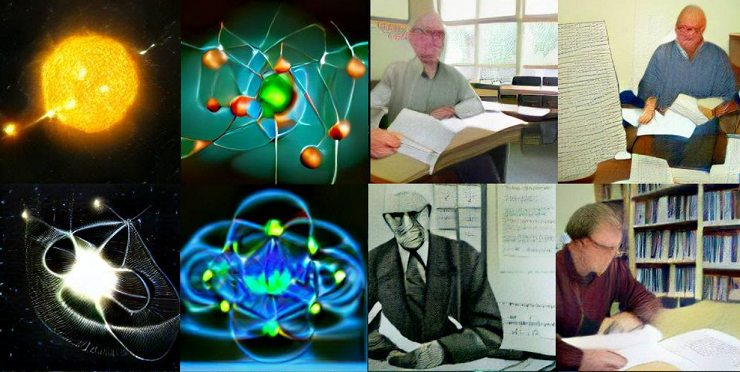For the most part, symbolic regression has been a finicky and computationally intensive machine learning method, garnering far less attention than deep learning in general over the past decade or so.

In part this has been due to the use-it-and-lose-it approach of genetic or probabilistic methods, which have to start from scratch for each new dataset, a characteristic shared with intermediate applications of deep learning to symbolic regression like AI Feynman.

The use of transformers as integral components in symbolic regression has allowed recent models to take advantage of large-scale pre-training, with a consequent decrease in energy, time, and computational hardware requirements at inference time.

This trend was further extended by new models that can estimate numerical constants as well as predict mathematical symbols, making for even faster inference and reportedly greater accuracy.

The task of generating symbolic expressions that can in turn be used to generate testable hypotheses is a very human one and stands at the core of science. Automated methods for symbolic regression have continued to make intriguing technical progress over the past two decades, but the real test is whether they can be useful to researchers doing real science.

Symbolic regression is starting to produce more and more publishable scientific results outside of technical demonstrations. A Bayesian approach to symbolic regression generated a new mathematical model for predicting cell division.

Another research team used a sparse regression model to generate plausible equations for ocean turbulence, paving the way for improved climate models at multiple scales.

A project combining graph neural networks and symbolic regression with Eureqa’s genetic algorithm recapitulated expressions describing multi-body gravitation and derived a new equation describing the distribution of dark matter from a conventional simulator.

### The Future of Symbolic Regression Algorithms

Symbolic regression is turning out to be a formidable tool in the scientist’s toolbox. The generalizing, scalable capabilities of transformer-based approaches are still hot-off-the-press and haven’t had time to trickle into general scientific practice. However, it promises to further empower scientific discovery as more researchers adapt and improve the models.

Many of these projects have been made available under permissive open-source licenses, so we can expect that they’ll have an impact in years, not decades, and their uptake may be much more widespread than proprietary software like Eureqa and TuringBot.

Symbolic regression is a natural complement to the often mysterious and notoriously difficult-to-interpret output of deep learning models, and the more understandable output in mathematical language can help generate new testable hypotheses and fuel intuitive leaps.

These characteristics and the outright capability of the latest generation of symbolic regression algorithms promise to yield quite a few opportunities for eureka moments.

Have any questions?
Contact Exxact Today!Deep Learning

## Neural-Symbolic Regression: Distilling Science from Data

The universe is noisy and confusing, complex enough to make predictions difficult. Human intelligence and intuition facilitate a basic understanding of some of the activities of the world around us. And they do so well enough to make basic sense of events at the macro space and time scales of the limited perspectives of individuals and small groups.

The natural philosophers of human prehistory and early history were mostly limited to common sense rationalization and guess and check. The limitations of these methods, especially for things that are just too big or too complex, are readily apparent in the prevalence and influence of superstition and magical thinking.

Not to disparage guessing and checking (it is the basis for the modern scientific method) but to see that a change in the human capability to investigate and understand was kindled by the desire and tools to distill physical phenomena into mathematical expressions.

This was especially evident after the time of Newton and others leading to the enlightenment, though there were traces of analytical reductionism in antiquity as well. The ability to go from observations to mathematical equations (and the predictions made possible by those equations) is an integral part of scientific exploration and progress.Deep learning is also fundamentally about learning transformations relating to input-output observations, just like human scientists attempting to learn functional relationships between inputs and outputs in the form of mathematical expressions.

The difference, of course, is that the input-output relationship learned by a deep neural network (a consequence of the universal approximation theorem) consists of an un-interpretable “black box” of numerical parameters, primarily weights, biases, and the nodes they connect.

The universal approximation theorem states that a neural network fulfilling very lenient criteria should be able to closely approximate any well-behaved function. In practice, neural networks are a brittle and leaky abstraction when representing input-output relationships arising from simple and precise underlying equations.

Neural networks tend to perform very poorly when making predictions outside of the distribution they were trained on unless special care is taken to train a model (or model ensemble) to predict uncertainty.

Deep learning predictions also don’t fare too well at making falsifiable predictions, i.e. the hypotheses making up the foundation of the scientific method, out of the box. So while deep learning is a well-proven tool adept at fitting data, its utility in arguably one of the most human pursuits of all, exploration of the universe around us via the scientific method, has been limited.

Despite the various shortcomings of deep learning in the human endeavor of science, we would be foolish to disregard the tremendous fitting power and numerous successes of deep learning in science-based disciplines.

Modern science generates tremendous amounts of data unfeasible for an individual (or even a small group) to look at the output and make intuitive leaps from noisy data to clean mathematical equations.

For this, we turn to symbolic regression, the automated or semi-automated method of reducing data to equations.

### The Current Gold Standard: Evolutionary MethodsBefore we get into some exciting recent research in applying modern deep learning to symbolic regression, we have to first visit the current state of the art of evolutionary methods for turning datasets into equations. The most commonly mentioned symbolic regression software package is Eureqa, based on genetic algorithms.

Eureqa was originally developed as a research project at Cornell University in Hod Lipson’s group, and made available as proprietary software from Nutonian, later acquired by DataRobot. Eureqa is integrated into the Datarobot platform, under Eureqa co-author and CTO of Datarobot Michael Schmidt.

Eureqa and similar symbolic regression tools use genetic algorithms to simultaneously optimize a population of equations for accuracy and simplicity.

TuringBot is an alternative symbolic regression package based on simulated annealing. Simulated annealing is an optimization algorithm analogous to the metallurgical annealing used to alter the physical properties of metals.

In simulated annealing, candidate solutions to an optimization problem are chosen with a decreasing “temperature,” where higher temperatures correspond to accepting worse solutions and are used to facilitate exploration early on, enabling the search for a global optimum and providing the energy to escape local optima.

TuringBot is available as a free version, but there are significant limits on dataset size and complexity and the code is not open for modification.

While commercial symbolic regression software (especially Eureqa) provides important baselines for comparison when developing new tools for symbolic regression, the utility of closed-source programs is limited.

An open-source alternative, called PySR, released under the Apache 2.0 license and led by Princeton Ph.D. student Miles Cranmer, shares the optimization objectives of accuracy and parsimony (simplicity) as well as combining methods used by Eureqa and TuringBot.

As well as providing a free and freely modifiable software library for performing symbolic regression, PySR is interesting from a software perspective: it is written in Python but uses the Julia programming language as a speedy backend.

While genetic algorithms are generally considered the current state-of-the-art for symbolic regression, there has been an exciting explosion of new symbolic regression strategies in the last few years.

Many of these new developments take advantage of the modern deep learning models, either as a function-approximation component in a multi-step process, or in an end-to-end manner based on large transformer models, originally developed for natural language processing, and anything in between.

In addition to new symbolic regression tools based on deep learning, there has been a resurgence in probabilistic and statistical methods as well, especially Bayesian statistics.

Combined with modern computational capabilities, the new crop of symbolic regression software is not only interesting research in its own right but provides real utility and contribution to scientific disciplines embracing big datasets and comprehensive experiments.

### Symbolic Regression with Deep Neural Networks as Function Approximators

Thanks to the Universal Approximation Theorem, described and investigated by Cybenko and Hornik in the late 1980s/early 1990s, we can expect neural networks with at least one hidden layer of non-linear activations to be able to approximate any well-behaved mathematical function.

In practice, we tend to get much better performance with much deeper neural networks on more complex or complicated problems. However, in principle, a single hidden layer is all you need to approximate a wide range of functions.

The physics-inspired AI Feynman algorithm exploits the universal approximation theorem as one piece in a much more complex puzzle.

AI Feynman (and its successor, AI Feynman 2.0) were developed by physicists Silviu-Marian Udrescu and Max Tegmark (along with some colleagues). Reflecting the background of the authors, AI Feynman takes advantage of functional properties found in many physics equations, such as smoothness, symmetry, and compositionality along with a handful of others.

Neural networks come into play as function approximators, learning the input-output transform pair represented in a dataset (or “mystery,” as they call them) and facilitating the investigation of these properties by producing synthetic data under the same functional transform.

The functional properties AI Feynman leverages to solve problems are common in equations from physics but are not arbitrarily applied to the space of all possible mathematical functions. However, they are still reasonable assumptions to look for in a wide variety of functions corresponding to the real world.

As in the genetic algorithm and simulated annealing approaches described earlier, AI Feynman fits each new dataset from scratch. There is no generalization or pre-training involved and the deep neural networks make up only one carefully orchestrated piece of a much larger, physics-informed system.

AI Feynman symbolic regression made an impressive showing in deciphering a set of 100 equations (or mysteries) from The Feynman Lectures on Physics, but the lack of generalization means that each new dataset (corresponding to a new equation) requires a substantial computational budget.

A new cohort of deep learning strategies for symbolic regression utilize the wildly successful transformer model family, originally introduced as natural language models by Vaswani et al. These new approaches aren’t perfect, but the utilization of pre-training can make for substantial computational savings at inference time.

### First-Generation Symbolic Regression Based on Natural Language ModelsGiven the massive success of extremely large attention-based transformer models on diverse tasks in computer vision, audio, reinforcement learning, recommender systems, and many other domains (in addition to the original role of text-based natural language processing), it’s no surprise that transformer models would eventually be applied to symbolic regression as well.

While the domain of numerical input-output pairs to symbolic sequences requires some careful engineering, the sequence-based nature of mathematical expressions naturally lends itself to the transformer approach.

Crucially, the use of transformers for generating mathematical expressions enables them to take advantage of pre-training on the structure and numerical meaning of millions of automatically generated equations.

This also lays the foundations for improving models by scaling larger. Scaling is one of the principal advantages of deep learning, where larger models and more data continue to improve model performance far beyond the classical statistical learning limits of over-fitting.

Scaling was the principal advantage of a paper by Biggio et al. entitled “Neural-Symbolic Regression that Scales,” which we’ll refer to as NSRTS. The NSRTS transformer model uses a specialized encoder to cast each dataset of input-output pairs into a latent space. The encoded latent space has a fixed size, regardless of the input size to the encoder.

The NSRTS decoder then builds a sequence of tokens to represent an equation, conditioned on the encoded latent space and the symbols generated so far. Crucially, the decoder only outputs placeholders for numerical constants, but otherwise uses the same vocabulary as the pre-training equations dataset.

NSRTS uses PyTorch and PyTorch Lightning and is available under a permissive open-source MIT License.

Following the generation of a constant-free equation, referred to as an equation skeleton, NSRTS uses gradient descent to optimize constants. This approach, which layers a generic optimization algorithm on top of sequence generation, is shared by the so-called “SymbolicGPT,'' developed contemporaneously by Valipour et al.

Instead of an attention-based encoder as in the NSRTS approach, Valipour et al. used a model loosely based on Stanford’s point-cloud model, PointNet, to generate a fixed-dimension set of features for a transformer decoder to use for generating equations. Like NSRTs, SymbolicGPT uses BFGS to find numerical constants for equation skeletons that the transformer decoder generates.

### Second-Generation Symbolic Regression Based on Natural Language Models

While recent publications describe the use of NLP transformers to achieve generalization and scalability for symbolic regression, the models described above are not truly end-to-end as they don’t estimate numerical constants.

This can be a critical flaw: imagine a model that generates equations with 1000 sinusoidal bases of different frequencies. Optimizing the coefficient for each term with BFGS is likely to yield a very good fit to most input datasets, but in fact, it would just be a slow and roundabout way of performing Fourier analysis.

As recently as spring 2022, the second generation of transformer-based symbolic regression models has been published on the ArXiv, in the SymFormer by Vastl et al., and another end-to-end transformer by Kamienny and colleagues.

The important difference between these and previous transformer-based symbolic regression models is that they predict numerical constants as well as sequences of symbolic math.

The SymFormer utilizes a dual-head transformer decoder to accomplish end-to-end symbolic regression. One head produces mathematical symbols and a second head learns the numerical regression task of estimating numerical constants wherever they appear in an equation.

The end-to-end models from Kamienny and Vastl differ in details such as the precision of their numerical estimates, but solutions from both groups still rely on a subsequent optimization step for refinement.

Even so, according to the claims of the authors, they have faster inference times and produce more accurate results than previous methods, producing better equation skeletons and giving refining optimization steps good starting points with estimated constants.

### Symbolic Regression Comes of AgeFor the most part, symbolic regression has been a finicky and computationally intensive machine learning method, garnering far less attention than deep learning in general over the past decade or so.

In part this has been due to the use-it-and-lose-it approach of genetic or probabilistic methods, which have to start from scratch for each new dataset, a characteristic shared with intermediate applications of deep learning to symbolic regression like AI Feynman.

The use of transformers as integral components in symbolic regression has allowed recent models to take advantage of large-scale pre-training, with a consequent decrease in energy, time, and computational hardware requirements at inference time.

This trend was further extended by new models that can estimate numerical constants as well as predict mathematical symbols, making for even faster inference and reportedly greater accuracy.

The task of generating symbolic expressions that can in turn be used to generate testable hypotheses is a very human one and stands at the core of science. Automated methods for symbolic regression have continued to make intriguing technical progress over the past two decades, but the real test is whether they can be useful to researchers doing real science.

Symbolic regression is starting to produce more and more publishable scientific results outside of technical demonstrations. A Bayesian approach to symbolic regression generated a new mathematical model for predicting cell division.

Another research team used a sparse regression model to generate plausible equations for ocean turbulence, paving the way for improved climate models at multiple scales.

A project combining graph neural networks and symbolic regression with Eureqa’s genetic algorithm recapitulated expressions describing multi-body gravitation and derived a new equation describing the distribution of dark matter from a conventional simulator.

### The Future of Symbolic Regression Algorithms

Symbolic regression is turning out to be a formidable tool in the scientist’s toolbox. The generalizing, scalable capabilities of transformer-based approaches are still hot-off-the-press and haven’t had time to trickle into general scientific practice. However, it promises to further empower scientific discovery as more researchers adapt and improve the models.

Many of these projects have been made available under permissive open-source licenses, so we can expect that they’ll have an impact in years, not decades, and their uptake may be much more widespread than proprietary software like Eureqa and TuringBot.

Symbolic regression is a natural complement to the often mysterious and notoriously difficult-to-interpret output of deep learning models, and the more understandable output in mathematical language can help generate new testable hypotheses and fuel intuitive leaps.

These characteristics and the outright capability of the latest generation of symbolic regression algorithms promise to yield quite a few opportunities for eureka moments.

Have any questions?
Contact Exxact Today!